# 978-0134292380 Chapter 3

Document Type
Test Prep
Book Title
Fundamentals of Hydraulic Engineering Systems 5th Edition
Authors
A. Osman H. Akan, Ned H. C. Hwang, Robert J. Houghtalen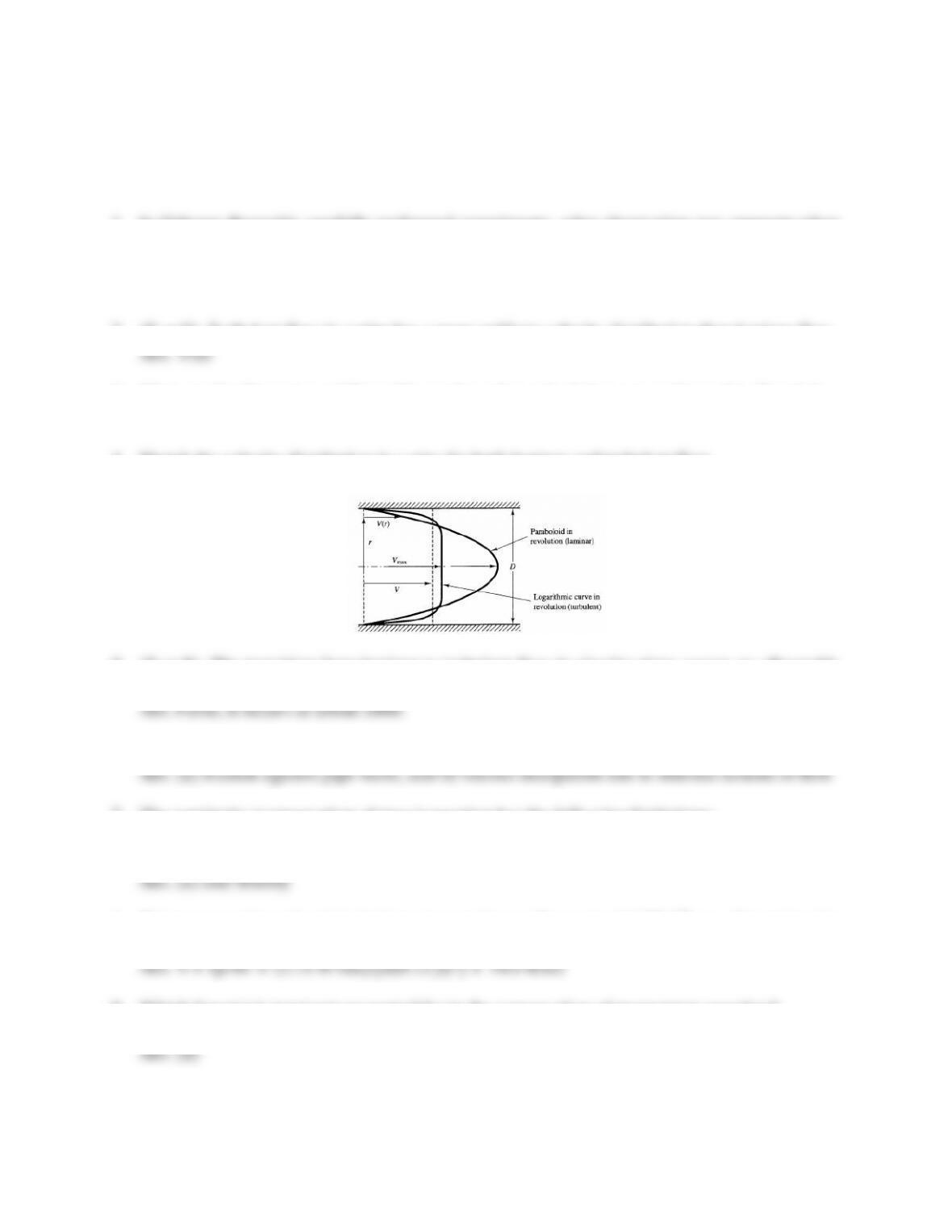18
TEST QUESTIONS & PROBLEMS - CHAPTER #3
1. In Osborne Reynolds carefully performed experiments, what observation was apparent when
laminar flow was occurring in the glass pipe?
Ans. When colored water was injected into the flow stream, it appeared as a straight line
extending to the downstream end, indicating laminar flow in the pipe.
2. (T or F) Turbulent flow in a pipe has a more uniform velocity distribution than laminar flow.
3. What are the dimensions of Reynolds number when calculations are performed in SI units?
Ans. Reynolds number is dimensionless.
4. Sketch the velocity distribution in a pipe for both laminar and turbulent flow.
Ans.
5. (T or F) The transition from laminar to turbulent flow in circular pipes occurs at a Reynolds
number of about 1000, but varies somewhat based on differences in experimental conditions
6. Water loses energy as it flows through a pipe. What causes most of the energy loss?
7. The continuity (conservation of mass) equation has the following limitations:
a) steady flow b) incompressible flow c) inviscid (negligible viscosity) flow
d) atmospheric pressure e) laminar flow f) turbulent flow
8. Water sprays through a 6-inch-diameter nozzle at a flow rate of 2.75 ft3/sec. Determine the
velocity of the water spray.
9. Which force(s) is (are) not accounted for in the conservation of momentum equation?
b) weight of fluid b) anchoring (wall) force c) pressure force d) surface tension force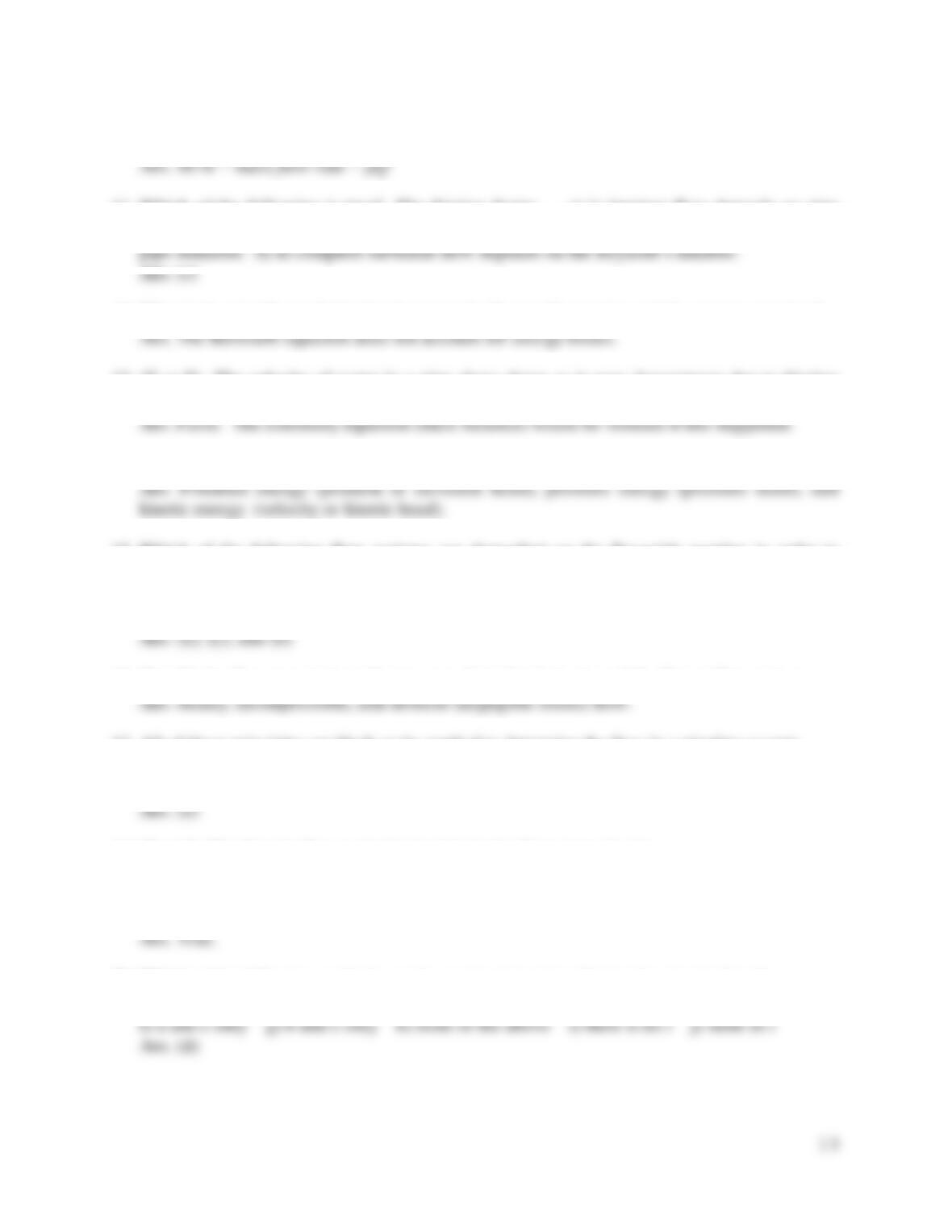19
10. In the derivation of the conservation of momentum equation, ∑F = (MV2 - MV1)/Δt became
∑F = ρQ(V2- V1)/Δt. Explain this step.
material. b) for turbulent flow depends on fluid pressure. c) in all flow regimes depends on
12. What is the significant distinction between the Bernoulli equation and the energy equation?
13. (T or F) The velocity of water in a pipe slows down as it goes downstream due to friction
and other losses.
14. Identify the three major forms of energy in pipe flow.
15. Which of the following flow regimes are dependent on the Reynolds number in order to
determine the friction factor?
a) laminar flow b) critical flow c) turbulent flow (smooth pipe)
d) turbulent flow (transition zone) e) turbulent flow (complete turbulence, rough pipes)
16. Identify the flow assumptions that were made in the derivation of the Bernoulli equation.
17. All of these principles are likely to be applied to determine the flow in a pipeline except:
a) conservation of momentum b) minor losses c) Darcy-Weisbach
d) Hazen-Williams e) energy balance f) conservation of mass (continuity)
18. (T or F) The Moody diagram in the book is in the SI system of units.
Ans. False. It is dimensionless.
19. (T or F) For laminar flow, the friction factor depends only on the Reynolds number.
20. Which of the following methods can be used to determine friction loss in pipelines?
a) Darcy-Weisbach b) Manning c) Hazen-Williams d) a, b, and c e) a and b only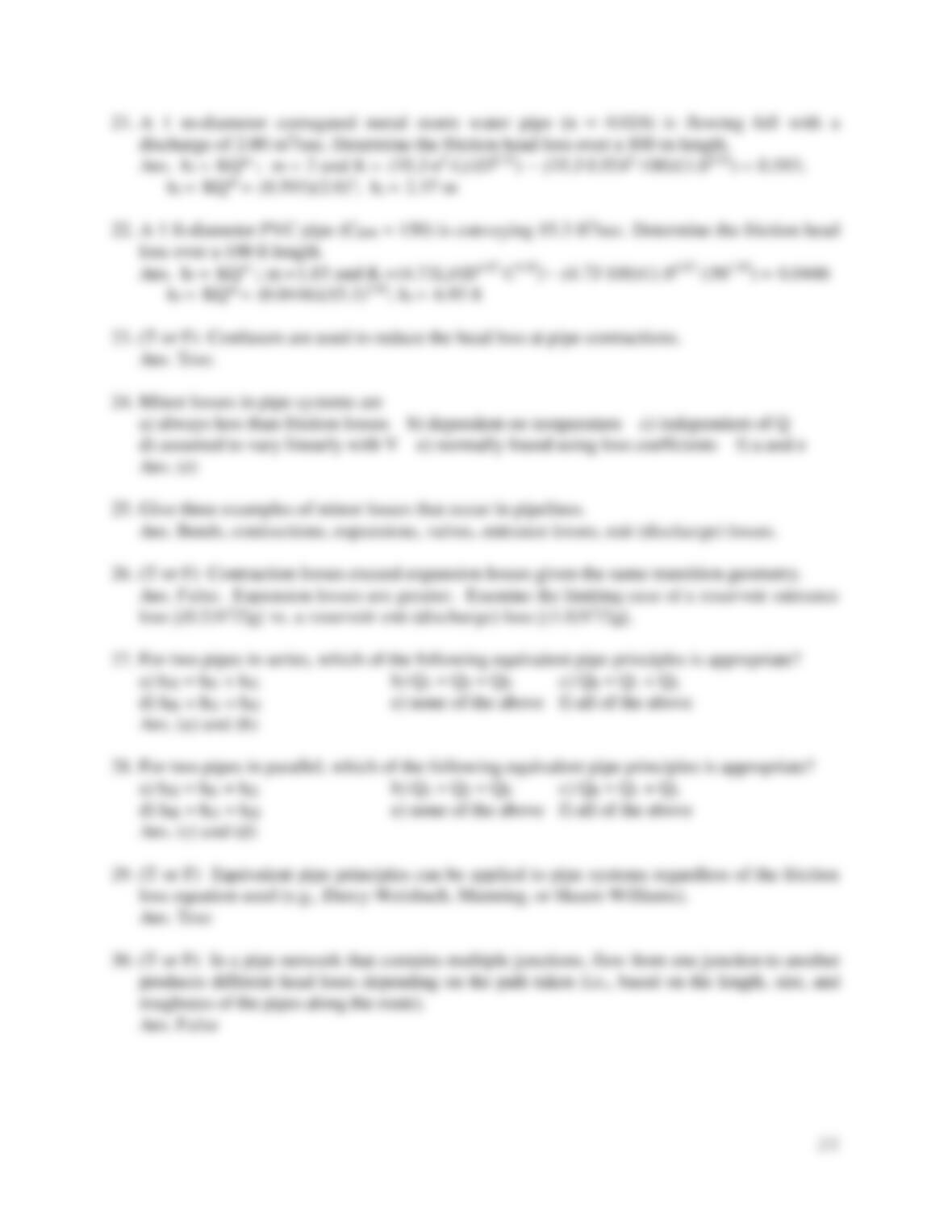21. A 1 m-diameter corrugated metal storm water pipe (n = 0.024) is flowing full with a
discharge of 2.00 m3/sec. Determine the friction head loss over a 100 m length.
22. A 1 ft-diameter PVC pipe (CHW = 150) is conveying 15.3 ft3/sec. Determine the friction head
loss over a 100 ft length.
23. (T or F) Confusors are used to reduce the head loss at pipe contractions.
24. Minor losses in pipe systems are
a) always less than friction losses b) dependent on temperature c) independent of Q
d) assumed to vary linearly with V e) normally found using loss coeffcients f) a and e
25. Give three examples of minor losses that occur in pipelines.
26. (T or F) Contraction losses exceed expansion losses given the same transition geometry.
27. For two pipes in series, which of the following equivalent pipe principles is appropriate?
a) hfE = hf1 + hf2 b) Q1 = Q2 = QE c) QE = Q1 + Q2
d) hfE = hf1 = hf2 e) none of the above f) all of the above
28. For two pipes in parallel, which of the following equivalent pipe principles is appropriate?
a) hfE = hf1 + hf2 b) Q1 = Q2 = QE c) QE = Q1 + Q2
d) hfE = hf1 = hf2 e) none of the above f) all of the above
29. (T or F) Equivalent pipe principles can be applied to pipe systems regardless of the friction
loss equation used (e.g., Darcy-Weisbach, Manning, or Hazen-Williams).
30. (T or F) In a pipe network that contains multiple junctions, flow from one junction to another
produces different head loses depending on the path taken (i.e., based on the length, size, and
roughness of the pipes along the route).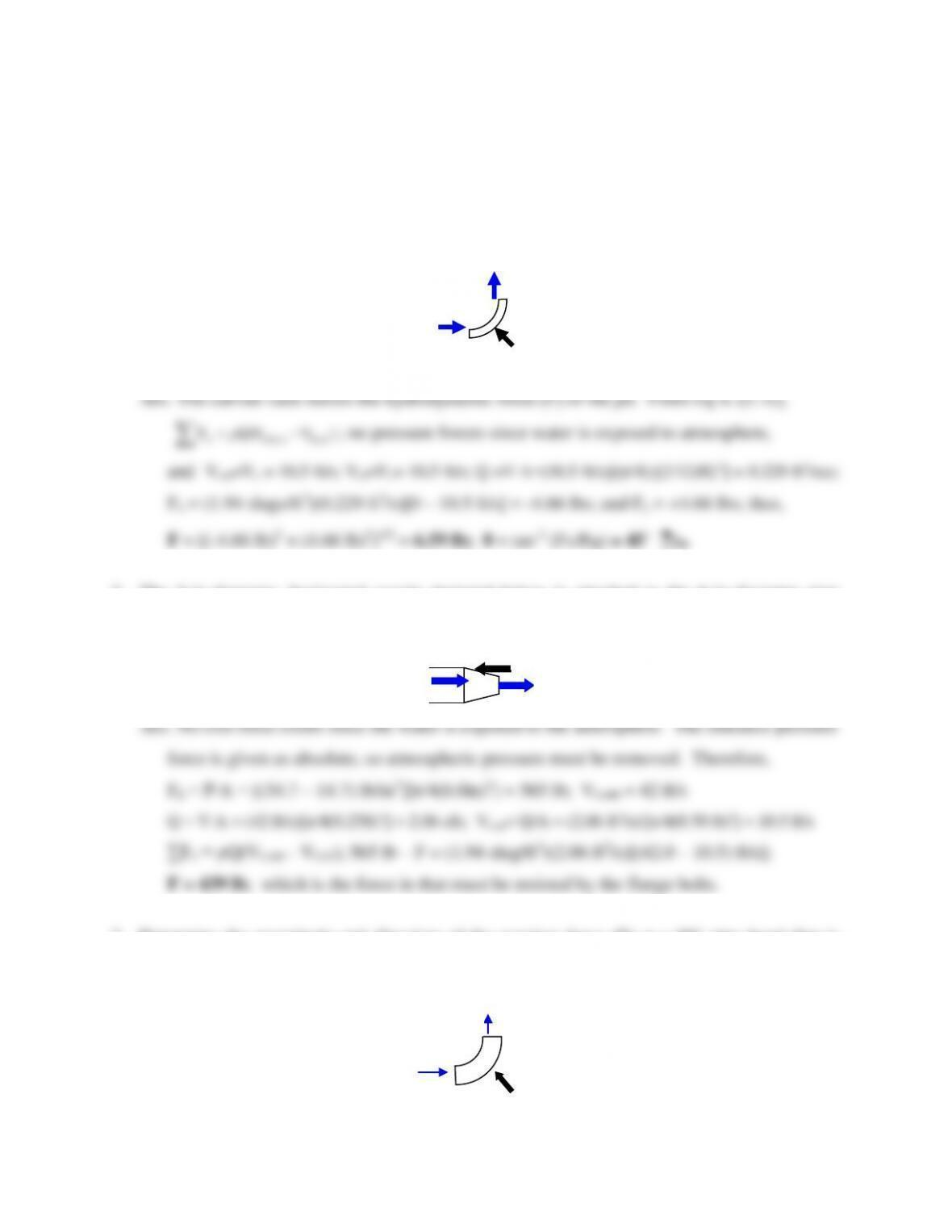21
Problems
1. A jet of water flowing freely in the atmosphere (x-direction) hits a curved vane and shoots
straight up (z-direction). The velocity of the water jet is 10.5 ft/sec and has a 2-in. diameter.
If the vane is assumed frictionless, determine the magnitude of the resultant force exerted on
the water jet by the vane and its direction.
2. The 3-in-diameter, horizontal nozzle depicted below is attached to the 6-in-diameter pipe
with flange bolts. The nozzle inlet pressure is 34.7 psi (absolute), and the exit velocity is 42
ft/sec. Compute the force in the flange bolts.
3. Determine the magnitude and direction of the reaction force (F) at a 90° pipe bend that is
passing a mass flow rate of 1,200 kg/sec. The diameter of the pipe is 70 cm and the pressure
head is 8.8 m just upstream of the bend and 8.6 m downstream of the bend.
Vin
Vout
F
Vin
Vout
F
V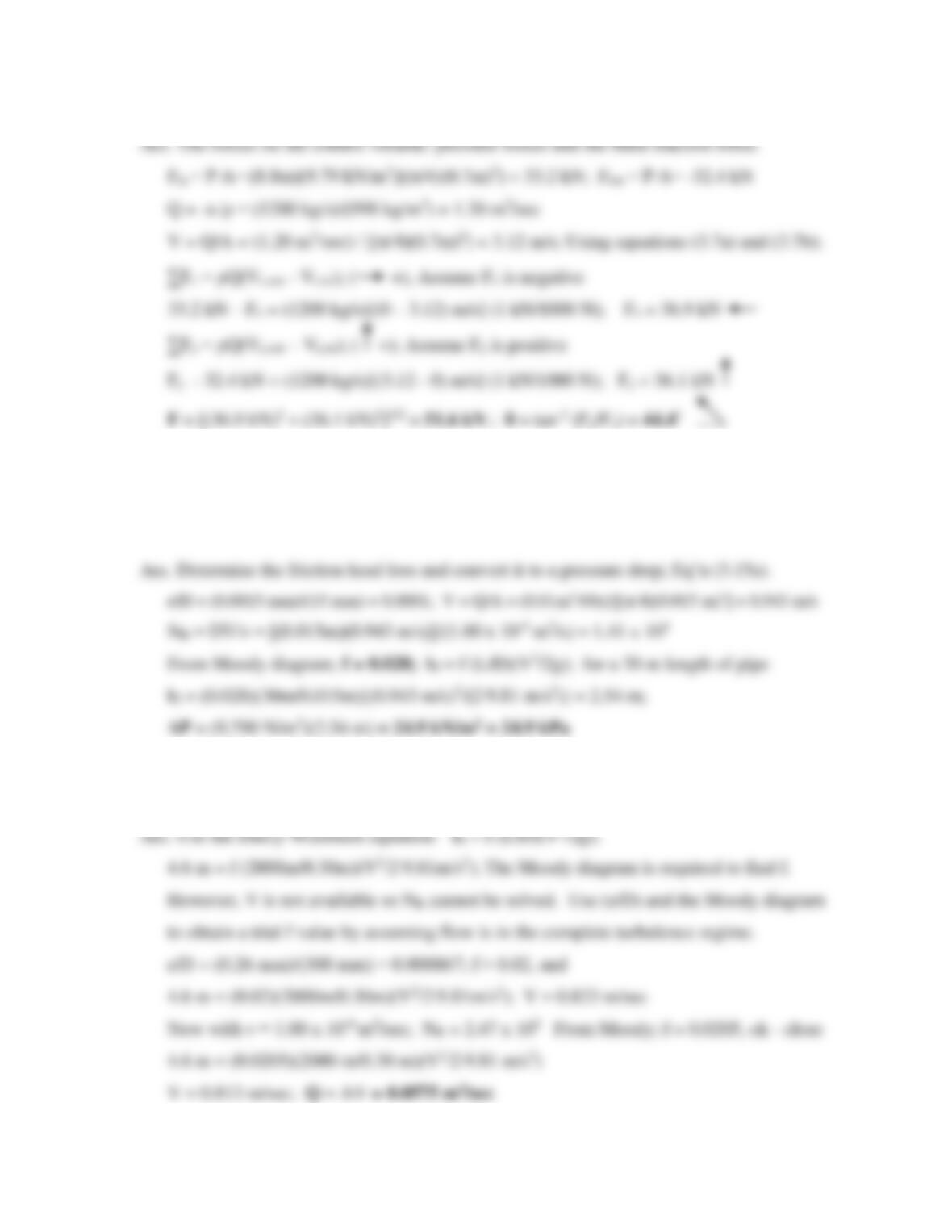22
4. A homeowner is interested in supplying water (20°C) to her wood shop that is about 30 m
from her house. However, she is concerned about the lack of water pressure. Calculate the
pressure drop she can expect for a flow rate of 10 liters per minute through a 1.5-cm copper
pipe. Assume minor losses are negligible.
5. A 30-cm diameter, cast-iron pipeline that is 2 km long carries water at 20°C. What is the
maximum discharge if a 4.6-m head loss is allowed?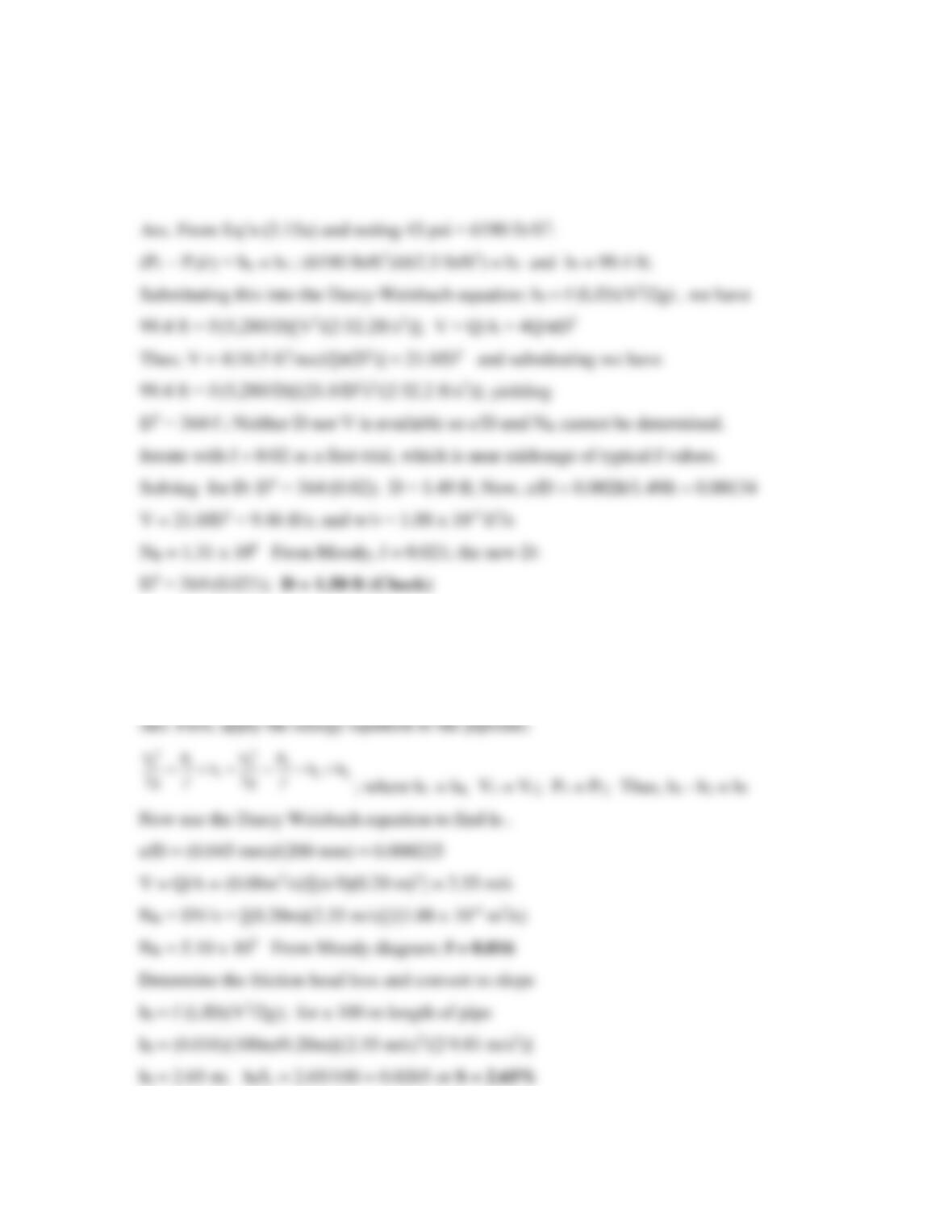23
6. A water main made of rough concrete loses 43 psi (lb/in.2) of pressure over a 1-mi horizontal
length. Estimate the size of the pipe required to carry 16.5 cfs (ft3/sec) of water at 68°F
(20˚C). Assume minor losses are negligible.
99.4 ft = f∙(5,280/D)[V2/(2∙32.2ft/s2)]; V = Q/A = 4Q/πD2
Thus; V = 4(16.5 ft3/sec)/[π(D2)] = 21.0/D2 and substituting we have
99.4 ft = f∙(5,280/D)[(21.0/D2)2/(2∙32.2 ft/s2)]; yielding
D5 = 364∙f ; Neither D nor V is available so e/D and NR cannot be determined.
Iterate with f = 0.02 as a first trial, which is near midrange of typical f values.
Solving for D: D5 = 364∙(0.02); D = 1.49 ft; Now, e/D = 0.002ft/1.49ft = 0.00134
V = 21.0/D2 = 9.46 ft/s; and w/ν = 1.08 x 10-5 ft2/s
NR = 1.31 x 106 From Moody, f = 0.021; the new D:
7. Water flows through a 20-cm commercial steel pipe at 20°C. The flow rate is 80 liters per
second and pressure is constant throughout the length of the pipe. Determine the slope of the
pipe. Assume minor losses are negligible.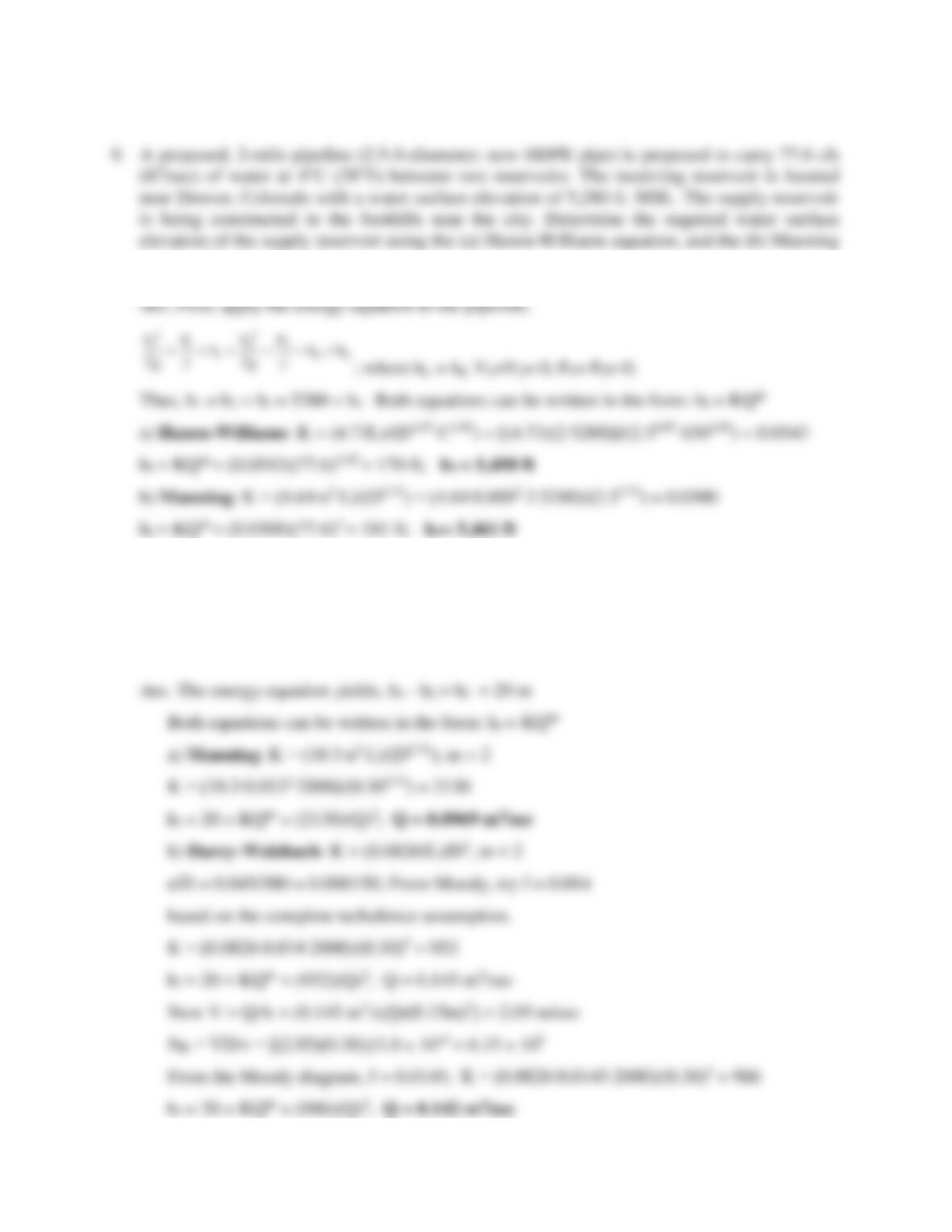24
8. A proposed, 2-mile pipeline (2.5-ft-diameter; new HDPE pipe) is proposed to carry 77.6 cfs
(ft3/sec) of water at 4°C (39˚F) between two reservoirs. The receiving reservoir is located
near Denver, Colorado with a water surface elevation of 5,280 ft. MSL. The supply reservoir
is being constructed in the foothills near the city. Determine the required water surface
equation? Ignore minor losses.
9. The elevation difference between two reservoirs 2000 m apart is 20 m. A 30-cm commercial
steel (CHW = 140) pipeline connects the reservoirs. The computed flowrate using the Hazen-
Williams equation is 0.136 m3/sec. Determine the flowrate using the Manning equation
(commercial steel, n = 0.013) and the Darcy-Weisbach equation (e = 0.045 mm).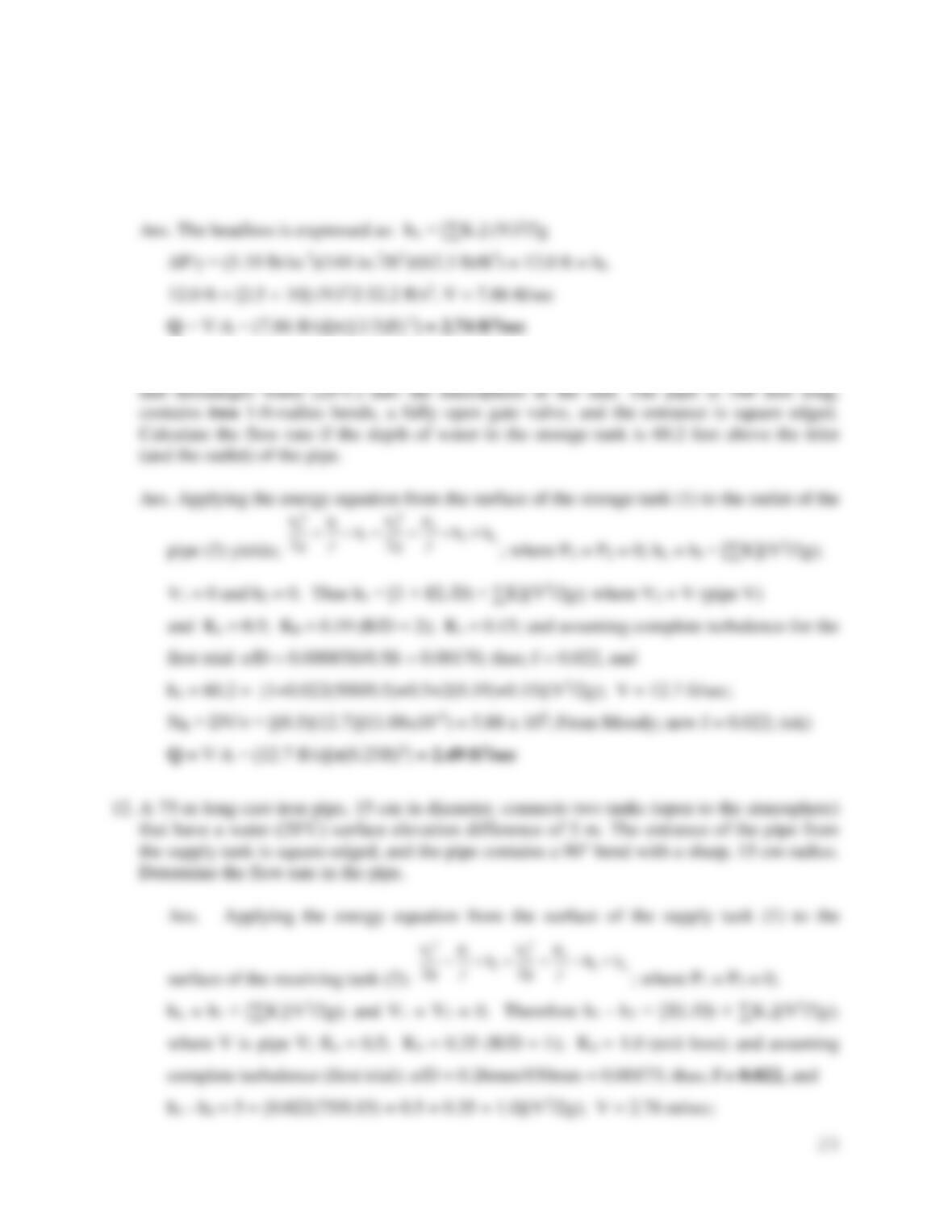25
10. A check valve (swing) and a globe valve are installed in series in an 8-in. pipe. Determine the
flow rate if the pressure drop across the valves is 5.19 psi (lbs/in.2). Assume that the friction
losses are negligible due to the short pipe length.
11. Water flows from the bottom of a storage tank through a horizontal, 6-inch pipe (cast iron)
12. A 75-m long cast-iron pipe, 15 cm in diameter, connects two tanks (open to the atmosphere)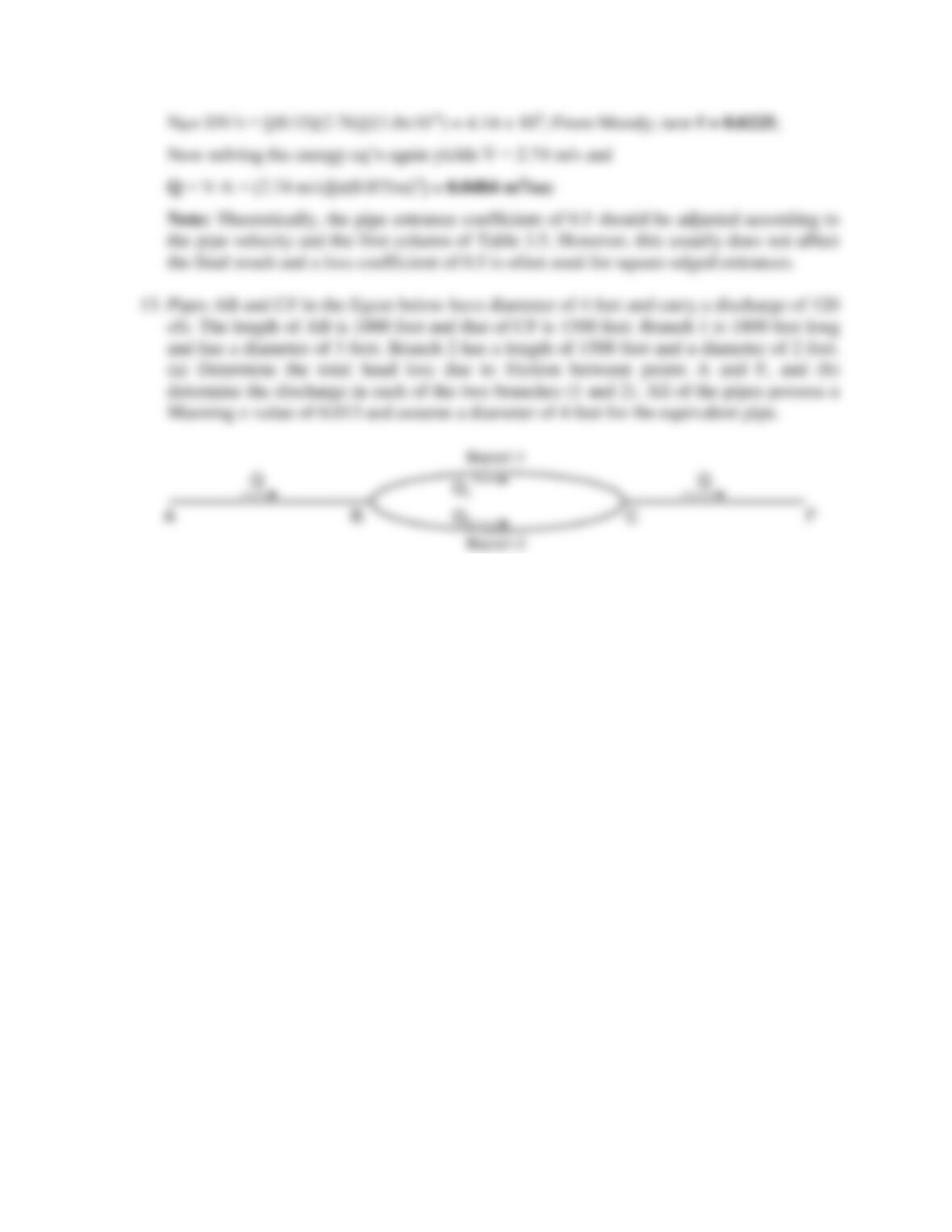## Trusted by Thousands ofStudents

Here are what students say about us.